# Euler summation method

(diff) ← Older revision | Latest revision (diff) | Newer revision → (diff)

One of the methods for summing series of numbers and functions. A series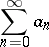(*)

is summable by means of the Euler summation method (-summable) to the sumifwhere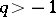and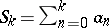.

The method was first applied by L. Euler for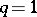to sum slowly-convergent or divergent series. Since the technique was later extended to arbitrary values ofby K. Knopp , it is also known for arbitraryas the Euler–Knopp summation method. This method is regular for(see Regular summation methods); if a series is-summable, then it is also-summable,, to the same sum (see Inclusion of summation methods). Forthe summability of the series (*) by the Euler summation method implies that the series is convergent. If the series is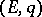-summable, then its terms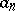satisfy the condition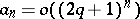,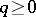. The Euler summation method can also be applied for analytic continuation beyond the disc of convergence of a function defined by means of a power series. Thus, the series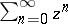is-summable to the sum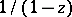in the disc with centre at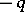and of radius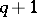.

How to Cite This Entry:
Euler summation method. Encyclopedia of Mathematics. URL: http://encyclopediaofmath.org/index.php?title=Euler_summation_method&oldid=19089
This article was adapted from an original article by I.I. Volkov (originator), which appeared in Encyclopedia of Mathematics - ISBN 1402006098. See original article